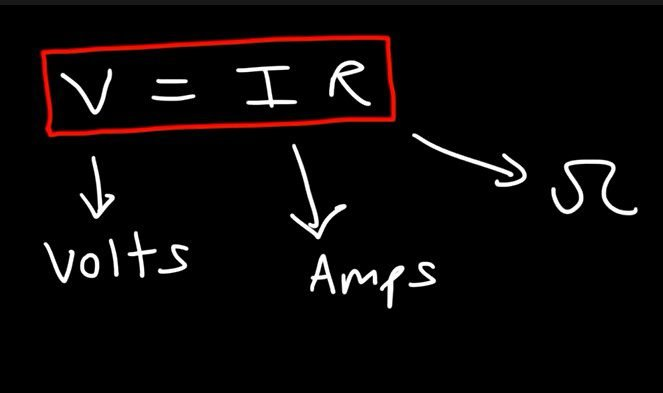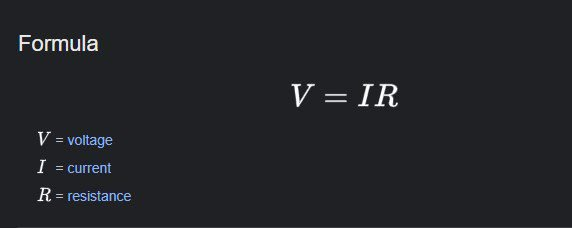# Ohm Law Formula in Electrical Circuits

Posted on

on this occasion we will share information about Ohm law formula for you so that your insight becomes wider. Here is the discussion, please read to the end

This article will discuss the Ohm law formula, of course complete with understanding to example questions and discussion.

Who here wants to be a reliable electronics engineer? If that is your goal, it means that you really have to understand the basic science , namely Ohm law. At school, you also learn this law , guys . Precisely in Physics subjects. But don’t worry, the Ohm law formula can be said to be easy, because it’s still basic. The important thing is that you understand the basics first, then deepen them to advanced levels, and become a reliable electronics engineer. Beuhh .. It’s really cool anyway. So that you understand about Ohm law, see the explanation below, come on !

## What is Ohm LawYep , so you don’t get confused when discussing the formula later, you must first understand the meaning of Ohm law itself. So, what is Ohm law , sis? Ohm law is a science in physics that states the relationship between electric current, voltage, and resistance in an electrical circuit.

Ohm law was first introduced by a German physicist named Georg Simon Ohm in 1827 through his work entitled ” The Galvanic Circuit Investigated Mathematically” . This is how Ohm law reads:

The amount of electric current (I) flowing through a conductor will be directly proportional to the voltage/potential difference (V) applied to it and inversely proportional to the resistance R

As already I mentioned earlier, if the law of ohm is the basis of a variety of science related electronic same electrical circuit. Such as TV sets, refrigerators, washing machines, and much more. So, yes it is true that Ohm law is the basis of all electrical circuits. That’s why you have to understand this material, guys .

## Ohm Law FormulaWell , after you understand the meaning and benefits of Ohm law, now you also understand the formula. Based on the sound of Ohm law in the previous point, the formula for Ohm law can be written as follows:

V = IR

Information:

• V : voltage (Volt)
• I : current strength (Amperes)
• R : resistance (Ω or Ohm)

The formula above can also be used to find the electric current and resistance. Stay behind and forth just formula, in accordance with the following pyramid, guys .

If you want to find the electric voltage, you use the formula V = IR. If it turns out that you need to find the electric current, then use the formula I = V/R. Well , if what you are looking for is electrical resistance, then use the formula R = V/I. The point is you need to remember the pyramid, yes, guys . It would be easier to remember, wouldn’t it?

## Sample Questions and Discussion

Okay, let’s just go straight to the example questions and discussion. Eitsss.. but before that, you need to prepare the writing utensil first! So, you can simply scribble deh so that more understand more about Ohm law.

Problems example:

In an electrical circuit, there is a power supply with a voltage of 20 Volts. The resistance in the circuit is 20 Ohms. Determine the current flowing in the circuit!

Discussion

Given: V = 20 Volts; R = 20 Ohms.